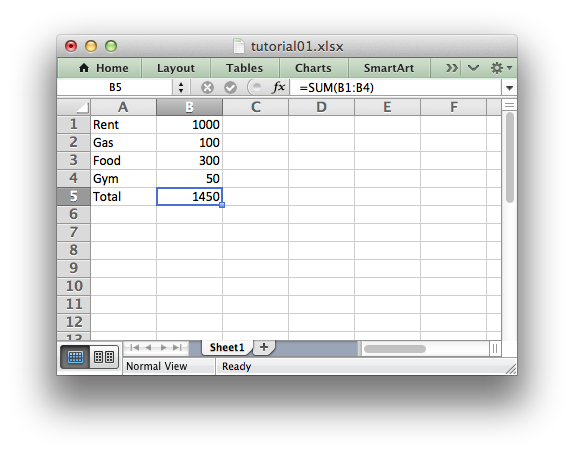libxlsxwriter
Tutorial 1: Create a simple XLSX file

Let's start by creating a simple spreadsheet using C and the `libxlsxwriter` library.

Say that we have some data on monthly outgoings that we want to convert into an Excel XLSX file:

Item Cost
Rent 1000
Gas 100
Food 300
Gym 50

To do that we can start with a small program like the following:

#include "xlsxwriter.h"
/* Some data we want to write to the worksheet. */
struct expense {
char item;
int cost;
};
struct expense expenses[] = {
{"Rent", 1000},
{"Gas", 100},
{"Food", 300},
{"Gym", 50},
};
int main() {
/* Create a workbook and add a worksheet. */
lxw_workbook *workbook = workbook_new("tutorial01.xlsx");
/* Start from the first cell. Rows and columns are zero indexed. */
int row = 0;
int col = 0;
/* Iterate over the data and write it out element by element. */
for (row = 0; row < 4; row++) {
worksheet_write_string(worksheet, row, col, expenses[row].item, NULL);
worksheet_write_number(worksheet, row, col + 1, expenses[row].cost, NULL);
}
/* Write a total using a formula. */
worksheet_write_string (worksheet, row, col, "Total", NULL);
worksheet_write_formula(worksheet, row, col + 1, "=SUM(B1:B4)", NULL);
/* Save the workbook and free any allocated memory. */
return workbook_close(workbook);
}

If we run this program we should get a spreadsheet that looks like this:This is a simple example but the steps involved are representative of all programs that use `libxlsxwriter`, so let's break it down into separate parts.

The first step is to include the header for the library:

#include "xlsxwriter.h"

Then we need some data to add to the spreadsheet. For the sake of this example we create and initialize some simple data structures. In a real application the input data might come from a database or a file.

struct expense {
char item;
int cost;
};
struct expense expenses[] = {
{"Rent", 1000},
{"Gas", 100},
{"Food", 300},
{"Gym", 50},
};

The next step is to create a workbook object in a `main` block or function using the workbook_new() function which takes the filename that we want to create:

lxw_workbook *workbook = workbook_new("tutorial01.xlsx");

The workbook object is then used to add a new worksheet via the workbook_add_worksheet() function:

If a `NULL` pointer is used for the worksheet name then a default name will be supplied using the Excel convention of `Sheet1`, `Sheet2`, etc. However we can also specify a name:

worksheet = workbook_add_worksheet(workbook, NULL ); // Defaults to Sheet1.
worksheet = workbook_add_worksheet(workbook, "Data"); // Data.
worksheet = workbook_add_worksheet(workbook, NULL ); // Defaults to Sheet3.

We can then use the worksheet object to write data via the worksheet_write_string() and worksheet_write_number() functions:

worksheet_write_string(worksheet, 0, 0, "Hello", NULL);
worksheet_write_number(worksheet, 1, 0, 1234.56, NULL);
Note
Rows and columns are zero indexed throughout the libxlsxwriter API. Thus, the first cell in a worksheet, `A1`, is equivalent to `(0, 0)`.

So in our example we iterate over our data and write it out as follows:

for (row = 0; row < 4; row++) {
worksheet_write_string(worksheet, row, col, expenses[row].item, NULL);
worksheet_write_number(worksheet, row, col + 1, expenses[row].cost, NULL);
}

We then add a formula to calculate the total of the items in the second column:

worksheet_write_formula(worksheet, row, col + 1, "=SUM(B1:B4)", NULL);

Finally, we close the Excel file via the close method:

return workbook_close(workbook);

And that's it. We now have a file that can be read by Excel and other spreadsheet applications.

In the next sections we will see how we can use the `libxlsxwriter` module to add formatting and other Excel features.

workbook_close
lxw_error workbook_close(lxw_workbook *workbook)
Close the Workbook object and write the XLSX file.
worksheet_write_formula
lxw_error worksheet_write_formula(lxw_worksheet *worksheet, lxw_row_t row, lxw_col_t col, const char *formula, lxw_format *format)
Write a formula to a worksheet cell.
workbook_new
lxw_workbook * workbook_new(const char *filename)
Create a new workbook object.
lxw_worksheet
Struct to represent an Excel worksheet.
Definition: worksheet.h:1603
lxw_workbook
Struct to represent an Excel workbook.
Definition: workbook.h:273
worksheet_write_string
lxw_error worksheet_write_string(lxw_worksheet *worksheet, lxw_row_t row, lxw_col_t col, const char *string, lxw_format *format)
Write a string to a worksheet cell.
worksheet_write_number
lxw_error worksheet_write_number(lxw_worksheet *worksheet, lxw_row_t row, lxw_col_t col, double number, lxw_format *format)
Write a number to a worksheet cell.